Courses

# Methods Of Transistor Biasing Electrical Engineering (EE) Notes | EduRev

## Electronic Devices

Created by: Cstoppers Instructors

## Electrical Engineering (EE) : Methods Of Transistor Biasing Electrical Engineering (EE) Notes | EduRev

The document Methods Of Transistor Biasing Electrical Engineering (EE) Notes | EduRev is a part of the Electrical Engineering (EE) Course Electronic Devices.
All you need of Electrical Engineering (EE) at this link: Electrical Engineering (EE)

METHODS OF TRANSISTOR BIASING:

1) Fixed bias (base bias)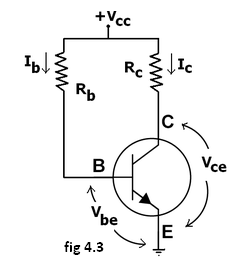This form of biasing is also called base bias. In the fig 4.3 shown, the single power source (for example, a battery) is used for both collector and base of a transistor, although separate batteries can also be used.

In the given circuit,

Vcc = IBRB + Vbe

Therefore,  IB = (Vcc - Vbe)/RB

Since the equation is independent of current ICR, dIB//dICR =0  and the stability factor is given by the equation….. reduces to

S=1+β

Since β is a large quantity, this is very poor biasing circuit. Therefore in practice the circuit is not used for biasing.

For a given transistor, Vbe does not vary significantly during use. As VCC is of fixed value, on selection of RB, the base current IB is fixed. Therefore this type is called fixed bias type of circuit.

Also for given circuit, VCC = ICRC + Vce

Therefore, Vce = VCC - ICRC

Merits:

• It is simple to shift the operating point anywhere in the active region by merely changing the base resistor (RB).

• A very small number of components are required.

Demerits:

• The collector current does not remain constant with variation in temperature or power supply voltage. Therefore the operating point is unstable.

• Changes in Vbe will change IB and thus cause RE to change. This in turn will alter the gain of the stage.

• When the transistor is replaced with another one, considerable change in the value of β can be expected. Due to this change the operating point will shift.

2) EMITTER-FEEDBACK BIAS:

The emitter feedback bias circuit is shown in the fig 4.4. The fixed bias circuit is modified by attaching an external resistor to the emitter. This resistor introduces negative feedback that stabilizes the Q-point. From Kirchhoff's voltage law, the voltage across the base resistor is

VRB = VCC - IERE - Vbe.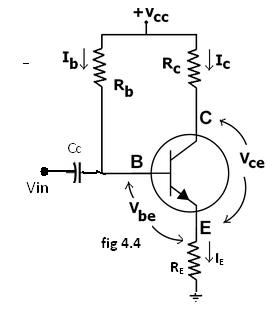From Ohm's law, the base current is

IB = VRB / RB.

The way feedback controls the bias point is as follows. If Vbe is held constant and temperature increases, emitter current increases. However, a larger IE increases the emitter voltage VE = IERE, which in turn reduces the voltage VRB across the base resistor. A lower base-resistor voltage drop reduces the base current, which results in less collector current because Ic = βIB. Collector current and emitter current are related by IC = α IE with α ≈ 1, so increase in emitter current with temperature is opposed, and operating point is kept stable.

Similarly, if the transistor is replaced by another, there may be a change in IC (corresponding to change in β-value, for example). By similar process as above, the change is neglected and operating point kept stable.

For the given circuit,

IB = (VCC - Vbe)/(RB + (β+1)RE).

Merits:

The circuit has the tendency to stabilize operating point against changes in temperature and β-value.

Demerits:

• In this circuit, to keep IC independent of β the following condition must be met: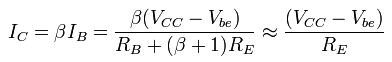which is approximately the case if ( β + 1 )RE >> RB.

• As β-value is fixed for a given transistor, this relation can be satisfied either by keeping RE very large, or making RB very low.

• If RE is of large value, high VCC is necessary. This increases cost as well as precautions necessary while handling.

• If RB is low, a separate low voltage supply should be used in the base circuit. Using two supplies of different voltages is impractical.

• In addition to the above, RE causes ac feedback which reduces the voltage gain of the amplifier.

COLLECTOR TO BASE BIAS  OR COLLECTOR FEED-BACK BIAS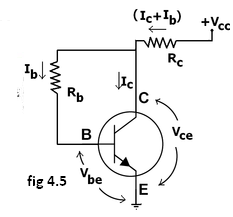This configuration shown in fig 4.5 employs negative feedback to prevent thermal runaway and stabilize the operating point. In this form of biasing, the base resistor Rb is connected to the collector instead of connecting it to the DC source VCC. So any thermal runaway will induce a voltage drop across the RC resistor that will throttle the transistor's base current.

From Kirchhoff's voltage law, the voltage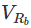across the base resistor Rb is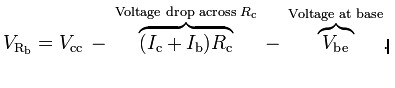By the Ebers–Moll model, Ic = βIb, and so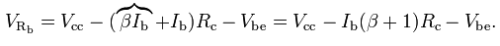From Ohm's law, the base currentand so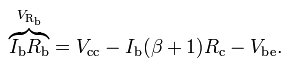Hence, the base current Ib is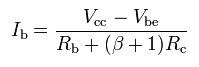If Vbe is held constant and temperature increases, then the collector current Ic increases. However, a larger Ic causes the voltage drop across resistor Rc to increase, which in turn reduces the voltage across the base resistor Rb. A lower base-resistor voltage drop reduces the base current Ib, which results in less collector current Ic. Since, Because increase in collector current with temperature is opposed, the operating point is kept stable.

Merits:

• Circuit stabilizes the operating point against variations in temperature and β (i.e. replacement of transistor)

Demerits:

• In this circuit, to keep Ic independent of β, the following condition must be met: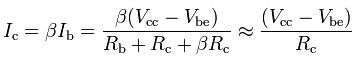which is the case when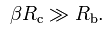• As β-value is fixed (and generally unknown) for a given transistor, this relation can be satisfied either by keeping Rc fairly large or making Rb very low.
• If Rc is large, a high Vcc is necessary, which increases cost as well as precautions necessary while handling.
• If Rb is low, the reverse bias of the collector–base region is small, which limits the range of collector voltage swing that leaves the transistor in active mode.
• The resistor Rb causes an AC feedback, reducing the voltage gain of the amplifier. This undesirable effect is a trade-off for greater Q-point stability.

Usage: The feedback also decreases the input impedance of the amplifier as seen from the base, which can be advantageous. Due to the gain reduction from feedback, this biasing form is used only when the trade-off for stability is warranted.

COLLECTOR –EMITTER FEEDBACK BIAS: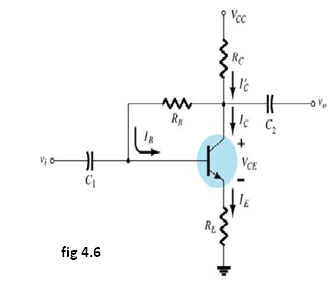The above fig 4.6 shows the collector –emitter feedback bias circuit that can be obtained by applying both the collector feedback and emitter feedback. Here the collector feedback is provided by connecting a resistance RB from the collector to the base and emitter feedback is provided by connecting an emitter RE from emitter to ground. Both feed backs are used  to control collector current and base current IB in the opposite direction to increase the stability as compared to the previous biasing circuits.

1. VOLTAGE DIVIDER BIAS OR SELF BIAS OR EMITTER BIAS:

The voltage divider as shown in the fig 4.7 is formed using external resistors R1 and R2. The voltage across R2 forward biases the emitter junction. By proper selection of resistors R1 and R2, the operating point of the transistor can be made independent of β. In this circuit, the voltage divider holds the base voltage fixed independent of base current provided the divider current is large compared to the base current. However, even with a fixed base voltage, collector current varies with temperature (for example) so an emitter resistor is added to stabilize the Q-point, similar to the above circuits with emitter resistor.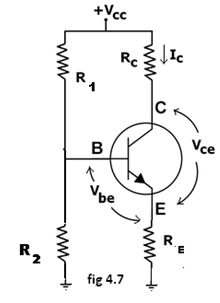In this circuit the base voltage is given by:

VB = voltage across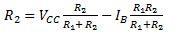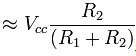Provided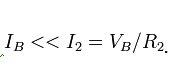Also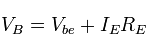For the given circuit,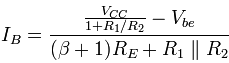Let the current in resistor R1 is I1 and this is divided into two parts – current through base and resistor R2. Since the base current is very small, so for all practical purposes it is assumed that I1 also flows through R2, so we have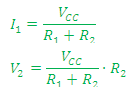Applying KVL in the circuit, we have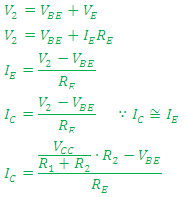It is apparent from above expression that the collector current is independent of transistor parameters thus the stability is excellent. In all practical cases the value of Vbe is quite small in comparison to the V2, so it can be ignored in the above expression. Hence, the collector current is almost independent of the transistor parameters thus this arrangement provides excellent stability.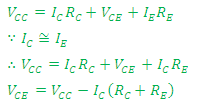The resistor RE provides stability to the circuit. If the current through the collector rises, the voltage across the resistor RE also rises. This will cause VCE to increase as the voltage V2 is independent of collector current. This decreases the base current, thus collector current increases to its former value.

Stability factor for such circuit arrangement is given by: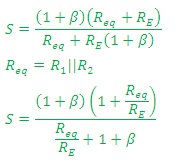If Req/RE is very small as compared to 1, it can be ignored in the above expression thus we have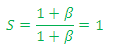which is excellent since it is the smallest possible value for the stability. In actual practice the value of stability factor is around 8-10, since Req/RE cannot be ignored as compared to 1.

Merits:

• Unlike above circuits, only one dc supply is necessary.
• Operating point is almost independent of β variation.
• Operating point stabilized against shift in temperature.

Demerits:

• In this circuit, to keep IC independent of β the following condition must be met: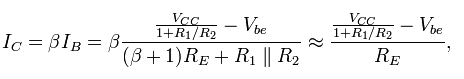which is approximately the case if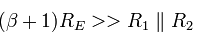where R1 || R2 denotes the equivalent resistance of R1 and R2 connected in parallel.

• As β-value is fixed for a given transistor, this relation can be satisfied either by keeping RE fairly large, or making R1||R2 very low.
• If RE is of large value, high VCC is necessary. This increases cost as well as precautions necessary while handling.
• If R1 || R2 is low, either R1 is low, or R2 is low, or both are low. A low R1 raises VB closer to VC, reducing the available swing in collector voltage, and limiting how large RC can be made without driving the transistor out of active mode. A low R2 lowers Vbe, reducing the allowed collector current. Lowering both resistor values draws more current from the power supply and lowers the input resistance of the amplifier as seen from the base.
• AC as well as DC feedback is caused by RE, which reduces the AC voltage gain of the amplifier.

Usage: The circuit's stability and merits as above make it widely used for linear circuits.

The various biasing circuits considered use some type of negative feedback to stabilize the operation point. Also, diodes, thermistors and sensistors can be used to compensate for variations in current.

Offer running on EduRev: Apply code STAYHOME200 to get INR 200 off on our premium plan EduRev Infinity!

## Electronic Devices

53 videos|56 docs|97 tests

,

,

,

,

,

,

,

,

,

,

,

,

,

,

,

,

,

,

,

,

,

;